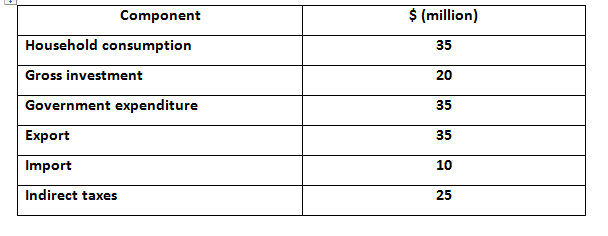Aug 12, 2023

QUESTION 1

1. Illustrate and explain the Gross Domestic Product (GDP) and unemployment for Malaysia for the years 2018-2021 using graphs.
2. Identify the macroeconomic problems that Malaysia is currently facing and discuss how the Malaysian government and Bank Negara Malaysia can mitigate the identified macroeconomic problems.

QUESTION 2

Given that information for Country A are as below:a) Calculate the GDP market price for Country A

b) Calculate the GDP factor cost for Country A

C) Discuss the problems in calculating Gross Domestic Product (GDP) for a country

QUESTION 3

Given:     C = 600 + 0.8Yd                  I = 500           G = 300                  T=200

a) Determine the equilibrium level of income using expenditure and injection-leakage approach

b) Calculate the value of the multiplier for this economy

Calculate the equilibrium level of income when there is an increase in investment from 500 to 800 using expenditure and injection-leakage approach.

Discuss the differences between expansionary fiscal policy and contractionary fiscal policy.